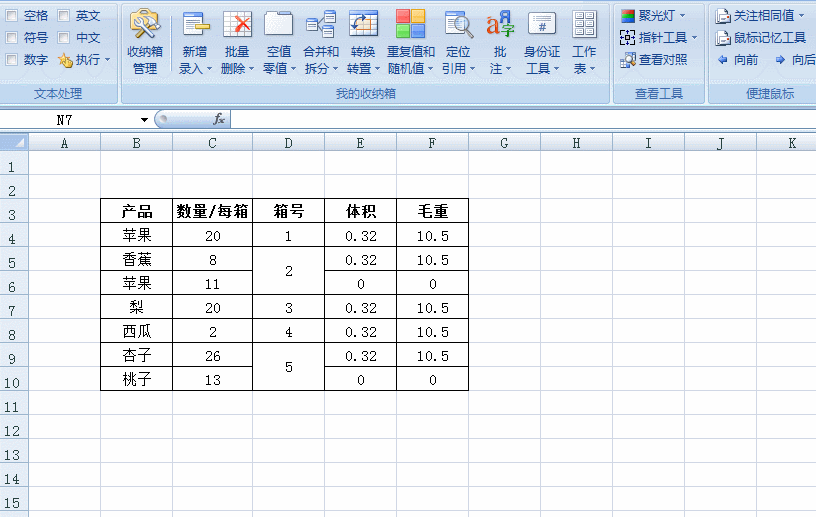`等级:学者1.我们需要先选中一个区域（只允许一列）

2.接着输入我们要合并的其他列的列号，VBA代码类似：Application.InputBox(prompt:="输入要合并的列名(用逗号隔开，如 E,F,G):", Type:=2,Default:="I,J,K,M,N,O")

3.循环输入的列号，依次合并。

1.用户有可能会选择整列，这时候如果使用for 循环，Excel将会卡死。所以我们必须检查选中区域的大小。（以下的VBA代码还缺少这个判断）

VBA代码如下：

`Sub 应用选区的合并格式到其他列()    On Error GoTo l_err        Dim r As Range    Dim i, n As Integer, beginRow As Integer    Dim cols As String    Dim arr() As String, colTgt As String        If Selection.Columns.Count > 1 Then        MsgBox "选区不允许包含多个列！"        Exit Sub    End If    cols = Application.InputBox(prompt:="输入要合并的列名(用逗号隔开，如 E,F,G):", Type:=2,Default:="I,J,K,M,N,O")    arr = Split(cols, ",")        Application.DisplayAlerts = False        For Each r In Selection        If r.MergeCells Then            If r.MergeArea.Columns.Count = 1 Then   '合并方向：单列                If r.MergeArea.Cells.Offset.Address = r.Address Then                    n = r.MergeArea.Count                    beginRow = r.MergeArea.Cells.Offset.Row                                        For i = 0 To UBound(arr)                        colTgt = arr(i)                        Range(colTgt & beginRow & ":" & colTgt & CStr(beginRow + n - 1)).Merge                    Next i                End If            End If        End If    Next        Application.DisplayAlerts = True    Exit Subl_err:    Application.DisplayAlerts = True    MsgBox "发生错误：" & Err.DescriptionEnd Sub`等级:传说级人物